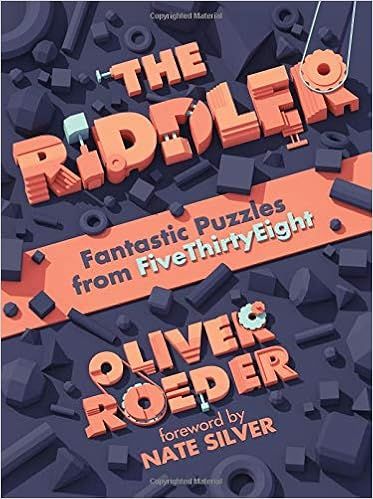# take a random integerA weird puzzle from FiveThirtyEight: what is the probability that the product of three random integers is a multiple of 100? Ehrrrr…, what is a random integer?! The solution provided by the Riddler is quite stunning

Reading the question charitably (since “random integer” has no specific meaning), there will be an answer if there is a limit for a uniform distribution of positive integers up to some number . But we can ignore that technicality, and make do with the idealization that since every second, fourth, fifth, and twenty-fifth integer are divisible by and , the chances of getting a random integer divisible by those numbers are , , , and .

as it acknowledges that the question is meaningless, then dismisses this as a “technicality” and still handles a Uniform random integer on {1,2,…,N} as N grows to infinity! Since all that matters is the remainder of the “random variable” modulo 100, this remainder will see its distribution vary as N moves to infinity, even if there is a 100 or so periodicity…